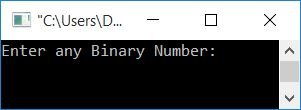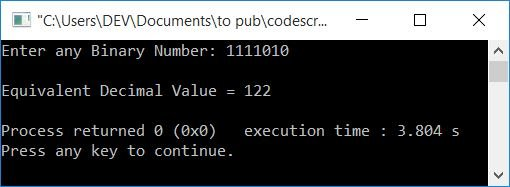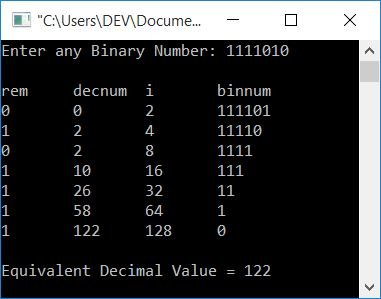# C++ Program to Convert Binary to Decimal

In this article, you will learn and get code on binary to decimal conversion with and without using functions in C++.

But before going through the program, if you are not aware of the steps, formula, or logic used behind the conversion of binary to decimal, you can refer to the section on binary to decimal conversion. You will learn everything there in a very short period of time.

## Binary to Decimal without a Function in C++

To convert any number entered by the user (in the binary number system) to its equivalent decimal value in C++ programming, you have to ask the user to enter the binary number first. And then apply the logic and convert it into its equivalent decimal value, as shown in the program given below.

Let's have a look at the program first; I will explain it later on.

```#include<iostream>
using namespace std;
int main()
{
int binnum, decnum=0, i=1, rem;
cout<<"Enter any Binary Number: ";
cin>>binnum;
while(binnum!=0)
{
rem = binnum%10;
decnum = decnum + (rem*i);
i = i*2;
binnum = binnum/10;
}
cout<<"\nEquivalent Decimal Value = "<<decnum;
cout<<endl;
return 0;
}```

This program was built and runs under the Code::Blocks IDE. Here is its sample run:Now supply any input as a binary number, say 1111010, and press the ENTER key to see its equivalent value in decimal, as shown in the output given below:#### Program explained with a dry run

The dry run of the above program with user input 1111010 goes in such a way that:

1. decnum=0, i=1, and binnum=1111010 (entered by the user) are the initial values.
2. The condition binnum!=0 (inside the while loop) or 1111010!=0 evaluates to be true.
3. Therefore, program flow goes inside the loop.
4. And binnum%10, 1111010%10, or 0 is assigned to rem. Now rem=0
5. Similarly, decnum+(rem*i) or 0+(0*1) or 0 gets initialized to decnum. Now decnum=0
6. After that, i*2 or 1*2 or 2 is initialized to i. Now i=2
7. And at last, binnum/10 or 1111010/10 or 111101 gets initialized to binnum. Now binnum=111101
8. After executing all four statements of the while loop (for the first time), we have decnum=0, i=2, and binnum=111101.
9. Program flow goes back to the condition of the while loop.
10. Again, the condition binnum!=0 or 111101!=0 is evaluated to be true, therefore the program flow again goes inside the loop.
11. And binnum%10, 111101%10, or 1 is assigned to rem. Now rem=1
12. Similarly, decnum+(rem*i) or 0+(1*2) or 2 gets initialized to decnum. Now decnum=2
13. And i*2 or 2*2 or 4 is assigned to i. Now i=4
14. And at last, binnum/10 or 111101/10 or 11110 gets initialized to binnum. Now binnum=11110
15. Now process from step no. 9 to 14 with the new values of decnum, i, and binnum.
16. decnum=2, i=8, and binnum=1111 are now our values.
17. Go to step no. 15.
18. Now we have decnum=10, i=16, and binnum=111.
19. Go to step no. 15.
20. Now we have decnum=26, i=32, and binnum=11.
21. Go to step no. 15.
22. Now we have decnum=58, i=64, and binnum=1.
23. Go to step no. 15.
24. Now we have decnum=122, i=128, and binnum=0.

#### Dry Run in Tubular Form

while loop evaluation rem decnum i binnum
1st Evaluation 0 0 2 111101
2nd Evaluation 1 2 4 11110
3rd Evaluation 0 2 8 1111
4th Evaluation 1 10 16 111
5th Evaluation 1 26 32 11
6th Evaluation 1 58 64 1
7th Evaluation 1 122 128 0

You can also print the value of all four variables, say, rem, decnum, i,, and binnum, during the execution of the while loop by placing the following statement:

`cout<<"\nrem\tdecnum\ti\tbinnum\n";`

just before the while loop. And the following statement:

`cout<<rem<<"\t"<<decnum<<"\t"<<i<<"\t"<<binnum<<endl;`

just after the fourth statement of while loop's body. Now, with user input of 1111010, output looks like this:## Binary to decimal conversion using a function in C++

This program converts binary to decimal using a user-defined function, BinToDec(). It takes a binary number as its argument and returns its equivalent decimal value.

This program uses the pow() function. It takes two arguments; the first argument is known as the base, whereas the second is known as the exponent. Therefore, pow(2, 5) means 25, which equals 32.

```#include<iostream>
#include<math.h>
using namespace std;
int BinToDec(int bin);
int main()
{
int binnum, decnum;
cout<<"Enter any Binary Number: ";
cin>>binnum;
decnum = BinToDec(binnum);
cout<<"\nEquivalent Decimal Value = "<<decnum;
cout<<endl;
return 0;
}
int BinToDec(int bin)
{
int dec=0, i=0, rem;
while(bin!=0)
{
rem = bin%10;
dec = dec + rem*pow(2,i);
i++;
bin = bin/10;
}
return dec;
}```

This program produces the same output as the previous program. You can check on your own with a dry run.

#### The same program in different languages

C++ Quiz

« Previous Program Next Program »